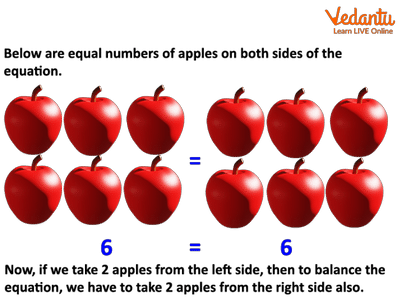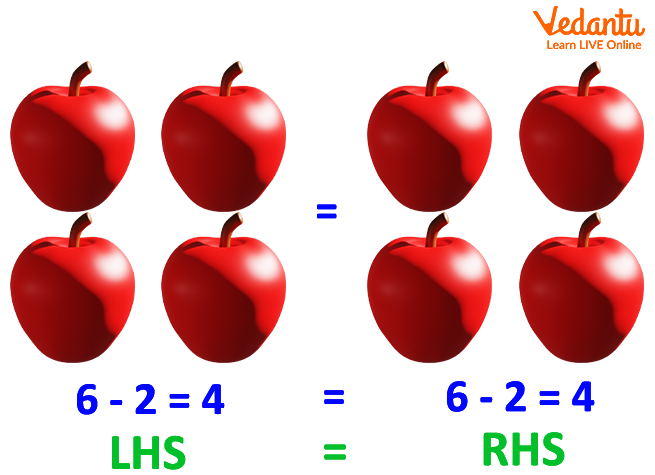Courses
Courses for Kids
Free study material
Free LIVE classes
More

# Know The Best Way To Balance The Equation With SubtractionLIVE
Join Vedantu’s FREE Mastercalss

## What Does Subtraction property of Equality Means In Mathmatics?

Are you looking to know “What does the subtraction property of equality hold”?

Yes!

Here, we will learn what the subtraction property of equality really means.

## What Is the Subtraction Property of Equality States?

The subtraction property of equality states that if we subtract the same number in both sides of the balanced equation, the equation will still be balanced and true.

Let us understand with an example.

## Subtraction Property of Equality Example

Below are equal numbers of apples on both sides of the equation.Equal Number of Apples

Now, if we take 2 apples from the left side, then to balance the equation, we have to take 2 apples from the right side also.Equal Number of Apples

## Subtraction Property of Equality Mathematical Equation

Let a, b, and c be any three real numbers.

If a = b

Then,

a – c = b – c

Therefore, the mathematical equation of subtraction property of equality is given as:

 a – c = b – c

## Finding an Unknown Variable Using the Subtraction Property of Equality

Now, we will learn how to find the value of an unknown variable using the subtraction property of equality.

To find the value of an unknown variable, we will use the inverse operation of addition or subtraction.

In other words, we will either add the same number on both sides of the equation or subtract the same number on both sides of the equation to balance the given equation.

Let us understand with an example.

Find the value of ‘x’ for the following equation:

x + 8 = 10

Solution:

The given equation is x + 8 = 10.

As 8 is added with the unknown variable ‘x’, we will subtract ‘8’ from both sides to balance the equation. Accordingly, the new equation will be:

x + 8 – 8 = 10 – 8 (Here, we have subtracted 8 from both sides because the inverse of addition is subtraction.)

x + 0 = 2

x = 2

Therefore, the value of x is 2.

## Subtraction Property of Equality Examples With Solutions

1. John has 20 balloons with him. He has 10 in one hand and 10 in another hand. If 3 balloons burst on one side, making him lose his balance. How many balloons should he burst from the other side to regain his balance?

Solution:

Here, we will use the subtraction property of equality to solve the question.

Total number of balloons John has = 20

Number of balloons in one hand = 10

Number of balloons in other hand = 10

10 = 10

Then, 3 balloons burst from one hand, resulting in the loss of balance. Therefore, the new equation will be:

10 – 3 = 7

To balance the other side of the equation, John will burst 3 ballons from the other hand also. Accordingly, the equation will be:

10 – 3 = 10 – 3

7 = 7

Now, the equation is balanced as both sides are equal. Therefore, LHS = RHS.

2. Find the value of y in y + 9 = 15 using the subtraction property of equality.

Solution:

As 9 is added with the unknown variable ‘x’, we will subtract ‘9’ from both sides to balance the equation. Accordingly, the new equation will be:

y + 9 = 15

y + 9 – 9 = 15 – 9 (inverse of addition is subtraction)

y = 6

Therefore, the value of y is 6.

In short, the subtraction property of equality states that if the same number is subtracted from both sides of the equation, then the equation remains balanced and true. For example, if you have 2 circles with 10 starts in each circle and if you take 2 stars from Circle 1, then you need to take 2 stars from Circle 2 also to balance the equation.

Last updated date: 28th Sep 2023
Total views: 140.4k
Views today: 3.40k

## FAQs on Know The Best Way To Balance The Equation With Subtraction

1. What does the term equality mean?

In Mathematics, the term equality defines the state of being equal. In other words, having the same expression, quantity, or value on the left side and right side of the equal sign. For example, one minute = 60 seconds. Equation is widely used in Mathematics for solving the equations in Algebra.

2. How do we represent equality in Mathematics?

In Mathematics, two things are said to be equal if they are the same in each and every aspect. This is why they have the same mathematical values and mathematical properties. We use the equal sign (=) to represent any two equal things. For example, the statement a = b implies that both a and b are equal in all aspects.

3. How the subtraction property of equality is used to compute the addition equation?

To use the subtraction property of equality when an equation is given with addition in it, simply subtract the same number or quantity from both sides of the equation to balance it.